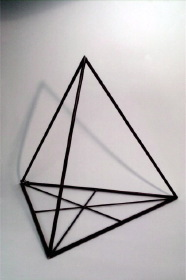Göttingen Collection of Mathematical Models and Instruments

# Tetrahedron

### Model 702Category: B II 27

### Description

Tetrahedron (brass pipe)

The tetrahedron has four equilateral triangles as faces. It has four vertices and six edges. At each vertex three triangles are meeting.

The tetrahedron is one of the five Platonic solids. A Platonic solid is a regular, convex polyhedron. The faces of a Platonic solid are congruent, regular polygons. At each vertex the same number of faces are meeting, see also model 411.

The five Platonic solids are Tetrahedron, Hexahedron (Cube), Octahedron, Dodecahedron and Icosahedron.

Showcase of this model is Case number 21

### References

Separataband M4 im Mathematischen Institut p. 231.

Separataband M5 im Mathematischen Institut p. 41, 45.

Separataband M5 im Mathematischen Institut p. 4.